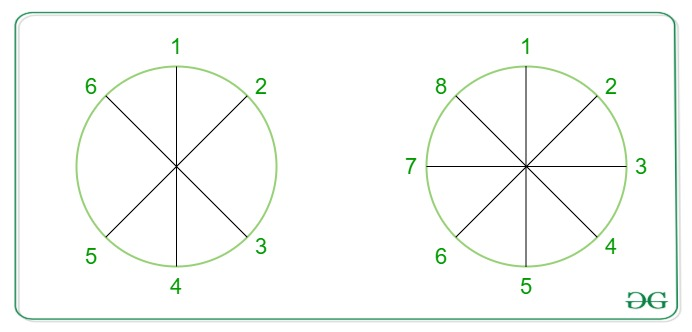Skip to content
Related Articles
Position of a person diametrically opposite on a circle
• Last Updated : 08 Jun, 2021

There are n people standing on the circumference of a circle. Given the position of a persom m, the task is to find the position of the person standing diametrically opposite to m on the circle.Examples:

Input: n = 6, m = 2
Output:
Position 5 is opposite to 2 when there are 6 positions in total
Input: n = 8, m = 5
Output:

Approach: There are two cases:

• If m > n / 2 then answer will always be m – (n / 2).
• If m ≤ n / 2 then answer will always be m + (n / 2).

Below is the implementation of the above approach:

## C++

 `// C++ implementation of the approach``#include ``using` `namespace` `std;`` ` `// Function to return the required position``int` `getPosition(``int` `n, ``int` `m)``{``    ``if` `(m > (n / 2))``        ``return` `(m - (n / 2));`` ` `    ``return` `(m + (n / 2));``}`` ` `// Driver code``int` `main()``{``    ``int` `n = 8, m = 5;``    ``cout << getPosition(n, m);`` ` `    ``return` `0;``}`

## Java

 `// Java implementation of the approach ``class` `Sol``{`` ` `// Function to return the required position ``static` `int` `getPosition(``int` `n, ``int` `m) ``{ ``    ``if` `(m > (n / ``2``)) ``        ``return` `(m - (n / ``2``)); `` ` `    ``return` `(m + (n / ``2``)); ``} `` ` `// Driver code ``public` `static` `void` `main(String args[])``{ ``    ``int` `n = ``8``, m = ``5``; ``    ``System.out.println(getPosition(n, m)); `` ` `} ``}``// This code is contributed by Arnab Kundu`

## Python3

 `# Python3 implementation of the approach`` ` `# Function to return the ``# required position``def` `getPosition(n, m):`` ` `    ``if` `(m > (n ``/``/` `2``)) :``        ``return` `(m ``-` `(n ``/``/` `2``))`` ` `    ``return` `(m ``+` `(n ``/``/` `2``))`` ` `# Driver code``n ``=` `8``m ``=` `5``print``(getPosition(n, m))`` ` `# This code is contributed ``# by ihritik`

## C#

 `// C# implementation of the approach``using` `System;`` ` `class` `GFG``{``     ` `// Function to return the required position ``static` `int` `getPosition(``int` `n, ``int` `m) ``{ ``    ``if` `(m > (n / 2)) ``        ``return` `(m - (n / 2)); `` ` `    ``return` `(m + (n / 2)); ``} `` ` `    ``// Driver code ``    ``static` `public` `void` `Main ()``    ``{``         ` `    ``int` `n = 8, m = 5; ``    ``Console.WriteLine(getPosition(n, m)); ``    ``} ``}`` ` `// This code is contributed by ajit.`

## PHP

 ` (``\$n` `/ 2)) ``        ``return` `(``\$m` `- (``\$n` `/ 2));`` ` `    ``return` `(``\$m` `+ (``\$n` `/ 2));``}`` ` `// Driver code``\$n` `= 8;``\$m` `= 5;``echo` `getPosition(``\$n``, ``\$m``);`` ` `// This code is contributed``// by ihritik``?>`

## Javascript

 ``
Output:
`1`

Time Complexity: O(1)

Attention reader! Don’t stop learning now. Get hold of all the important mathematical concepts for competitive programming with the Essential Maths for CP Course at a student-friendly price. To complete your preparation from learning a language to DS Algo and many more,  please refer Complete Interview Preparation Course.

My Personal Notes arrow_drop_up